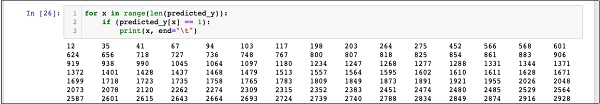# Logistic Regression in Python - Testing

We need to test the above created classifier before we put it into production use. If the testing reveals that the model does not meet the desired accuracy, we will have to go back in the above process, select another set of features (data fields), build the model again, and test it. This will be an iterative step until the classifier meets your requirement of desired accuracy. So let us test our classifier.

## Predicting Test Data

To test the classifier, we use the test data generated in the earlier stage. We call the predict method on the created object and pass the X array of the test data as shown in the following command −

```In : predicted_y = classifier.predict(X_test)
```

This generates a single dimensional array for the entire training data set giving the prediction for each row in the X array. You can examine this array by using the following command −

```In : predicted_y
```

The following is the output upon the execution the above two commands −

```Out: array([0, 0, 0, ..., 0, 0, 0])
```

The output indicates that the first and last three customers are not the potential candidates for the Term Deposit. You can examine the entire array to sort out the potential customers. To do so, use the following Python code snippet −

```In : for x in range(len(predicted_y)):
if (predicted_y[x] == 1):
print(x, end="\t")
```

The output of running the above code is shown below −The output shows the indexes of all rows who are probable candidates for subscribing to TD. You can now give this output to the bank’s marketing team who would pick up the contact details for each customer in the selected row and proceed with their job.

Before we put this model into production, we need to verify the accuracy of prediction.

## Verifying Accuracy

To test the accuracy of the model, use the score method on the classifier as shown below −

```In : print('Accuracy: {:.2f}'.format(classifier.score(X_test, Y_test)))
```

The screen output of running this command is shown below −

```Accuracy: 0.90
```

It shows that the accuracy of our model is 90% which is considered very good in most of the applications. Thus, no further tuning is required. Now, our customer is ready to run the next campaign, get the list of potential customers and chase them for opening the TD with a probable high rate of success.